# Question:determining if a function is isobaric in Maple

## Question:determining if a function is isobaric in Maple

Maple 2020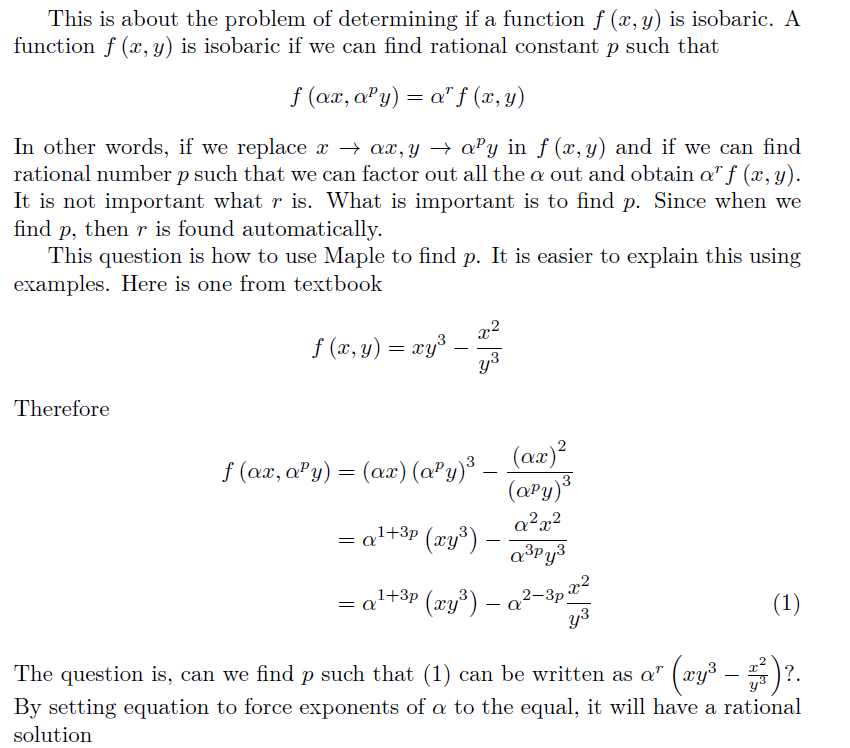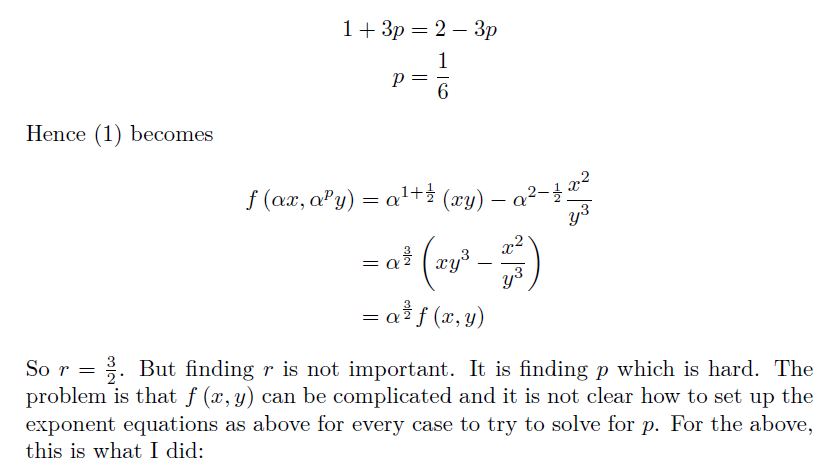```restart;
f:=(x,y)-> x*y^3-x^2/y^3;
eq:= subs([x=alpha*x,y=alpha^p*y],f(x,y));
lis:=[]:
for tmp in op(eq) do
if has(tmp,alpha) then
lis:=[op(lis),op(2,simplify(select(has,tmp,alpha)))];
fi;
od;
lis;
PDEtools:-Solve(lis=lis,p)

```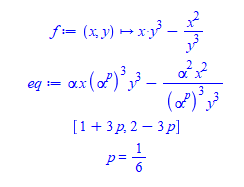This method happend to work for this example, but it does not work in general. This is because the function can be anything. Here is a second example

```restart;
f:=(x,y)-> -2/3*x+1/3*(x^2+3*y)^(1/2);
eq:= subs([x=alpha*x,y=alpha^p*y],f(x,y));

```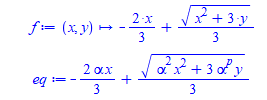We see by inspection if p=2 then we can write the above as, by setting p=2 as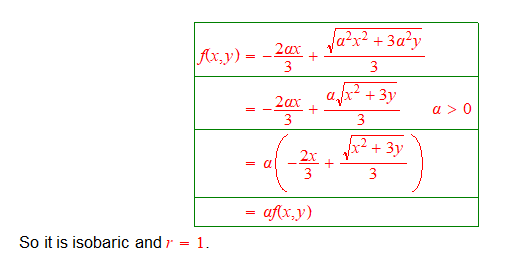But the Maple code I had above does not work on this

```restart;
f:=(x,y)-> -2/3*x+1/3*(x^2+3*y)^(1/2);
eq:= subs([x=alpha*x,y=alpha^p*y],f(x,y));
lis:=[]:
for tmp in op(eq) do
if has(tmp,alpha) then
lis:=[op(lis),simplify(select(has,tmp,alpha))];
fi;
od;
lis;
```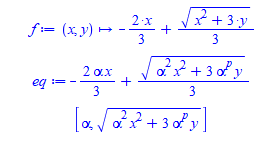The problem is finding all powers of alpha in each term and setting up an equation to find p such that all terms have same numerical value.

So I abandoned this method as too messy to program (it works well for hand solution, this is an example where solving something by hand is easier than on the computer) and then tried solve directly, like this (on the first example)

```restart;
f:=(x,y)-> x*y^3-x^2/y^3;
eq:= subs([x=alpha*x,y=alpha^p*y],f(x,y))- alpha^r*f(x,y)=0;
```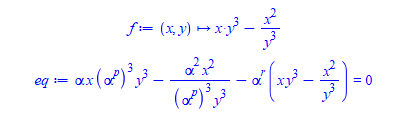And here is where I am stuck. I need to ask Maple if it can find alpha, r that are rational numbers, such that the above equation is solved.

`PDEtools:-Solve(eq,[p,r])`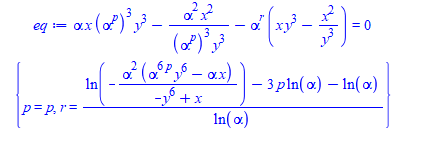But we know from above that p=1/6 and r=3/2 is solution

```simplify(subs([p=1/6,r=3/2],eq))
```

0=0

The question is: Can Maple solve such an equation for  p,r? I remember now reading something about parameteric solver in Maple. I need to look that up to see if it helps. I tried SolveTools:-SemiAlgebraic on this, but it did not help.

Are there better methods to determine in Maple if function is isobaric and to find the index p and r?

Reference: book

Notice, some places define isobaric function as one in which we can find p such that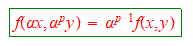But this is not correct. I've seen it on 2 pages on the net. The correct definition is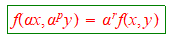Also note: When p=1 isobaric function becomes a homogeneous function which is special case of isobaric and r is now called the degree of homogeneity

But for isobaric, p do not have to be 1. This is the main difference.

f(x,y) will always be a function of x,y.  I will add more examples of isobaric functions to test against.

edit. Simplified question is restated below

After looking more into this. I found I actually wanted to solve the simpler problem, which isSo please ignore the general case of isobaric where r<>p-1 which is harder.

I only need to look at this case where r=p-1 which is actually simpler. It turned out this is what I need for my solver and not the general case.

I will now give my solution to this and show where the problem I am having.

I am using Maple solve to find p. So no r any more. Only solving for one variable. But Maple solve fails sometimes. And this is the problem.

The problem is that solve can find p for many cases, but fails on some, where there is clearly a solution. I will show 5 examples below.

```#example 1. WORKS
restart;
f:=(x,y)-> 3*sqrt(x*y);
eq:= subs([x=alpha*x,y=alpha^p*y],f(x,y))- alpha^(p-1)*f(x,y)=0;
PDEtools:-Solve(eq,p) assuming alpha>0;```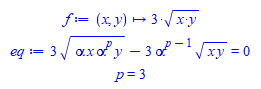```#example 2. WORKS
restart;
f:=(x,y)-> 4*(x*y)^(1/3);
eq:= subs([x=alpha*x,y=alpha^p*y],f(x,y))- alpha^(p-1)*f(x,y)=0;
PDEtools:-Solve(eq,p) assuming alpha>0;
```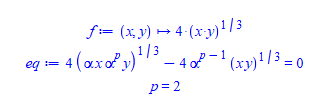```#example 3. WORKS
restart;
f:=(x,y)-> (-3*x^2*y-y^2)/(2*x^3+3*x*y);
eq:= subs([x=alpha*x,y=alpha^p*y],f(x,y))- alpha^(p-1)*f(x,y)=0;
PDEtools:-Solve(eq,p) assuming alpha>0;
```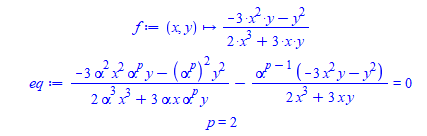```#example 4 WORKS
restart;
f:=(x,y)-> (-(x*y)/2+sqrt(x^2*y^2-4*y)/2)*y;
eq:= subs([x=alpha*x,y=alpha^p*y],f(x,y))- alpha^(p-1)*f(x,y)=0;
PDEtools:-Solve(eq,p) assuming alpha>0;

```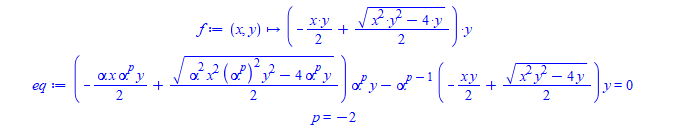And here is the one that does not work

```#Example 5. Does not work. How to make it work?
restart;
f:=(x,y)-> -2*x/3+sqrt(x^2+3*y)/3;
eq:= subs([x=alpha*x,y=alpha^p*y],f(x,y))- alpha^(p-1)*f(x,y)=0;
PDEtools:-Solve(eq,p) assuming alpha>0;

```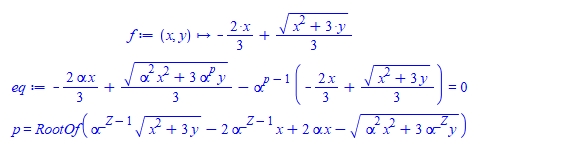There is a solution for this. p=2 works. Why solve did not find it? I mean, it gives RootOf, which does not help me decide if this is isobaric or not. Why it did not find the solution p=2?

`simplify(subs(p=2,eq)) assuming alpha>0`

0=0

Using Mathemtica, it found p=2 solution. How to make Maple find this solution is my question.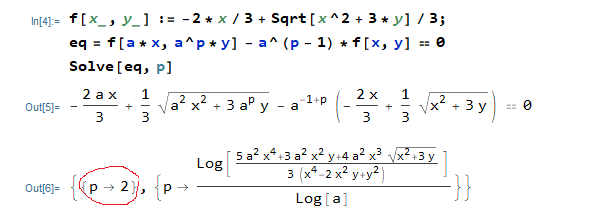Sorry for the long question. I first thought I needed the general isobaric case, but found later i needed the simpler one.﻿